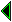## How much automation do we want?

We have seen that automation is possible even while producing an explicit proof, but how much do we actually want? Despite some tours de force by automated theorem provers, the emphasis in applications is moving increasingly towards interactive systems, where the user guides the prover. In mathematics this seems reasonable, since the proofs are important, and we don't usually want an ex cathedra assertion that something is true from a machine. On the other hand, we also don't want to have to direct proofs at very low levels, so some automation of 'obvious' steps is required.

As pointed out by John McCarthy, there is an important distinction between what is mathematically obvious and what is logically obvious. In mathematical proofs we want to skip over levels of reasoning which we can intuitively see to be trifling; but this intuition may be based on quite sophisticated domain-specific knowledge, and the step may not be at all obvious in the sense of having a formal proof which is easy to find using one of the common techniques for automated proof search (whether by machine or by hand). An interesting discussion of just what is logically obvious is given by [rudnicki-obvious]. It seems that we need more work on automating what is mathematically obvious, whereas existing technology is already capable of automating much larger logical steps than we can perceive as obvious, e.g. very large tautologies or even subtle predicate calculus reasoning like the following theorem due to \los:

(x y z. P(x,y)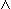P(y,z)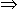P(x,z))(x y z. Q(x,y)Q(y,z)Q(x,z))(x y. Q(x,y)Q(y,x))(x y. P(x,y)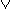Q(x,y))(x y. P(x,y))(x y. Q(x,y))

However, there are certain steps which are completely routine for a machine to check, and baffling to a human being, that we probably do want to automate, simply because carrying out the proof in detail is no more illuminating to the human than knowing, based on confidence in the correctness of the theorem prover, that it is valid. A good example is the following identity:

(x2 + y2 + z2 + w2) (x'2 + y'2 + z'2 + w'2) =
(x x' + y y' + z z' + w w')2 +
(x y' - y x' + w z' - z w')2 +
(x z' - z x' + y w' - w y')2 +
(x w' - w x' + z y' - y z')2

It's used as a lemma in the proof that every natural number is expressible as the sum of four squares. But proving it, at least simply by multiplying it out in a routine way, is no more illuminating than checking the result of a numerical calculation by hand. Of course a proof that exhibited a deep, simple reason for the above (e.g. something based on quaternions) is a different matter.

In any case, automating what is mathematically obvious is itself a research project in the artificial intelligence line. In the short term, it's probably better to accept a lower level of automation. As theorem-proving technology improves, it should be possible to simplify proofs automatically by excising intermediate steps which become unnecessary for the machine.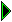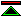John Harrison 1996/8/13; HTML by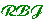1996/8/16